Ex 5.1

Chapter 5 Class 12 Continuity and Differentiability
Serial order wise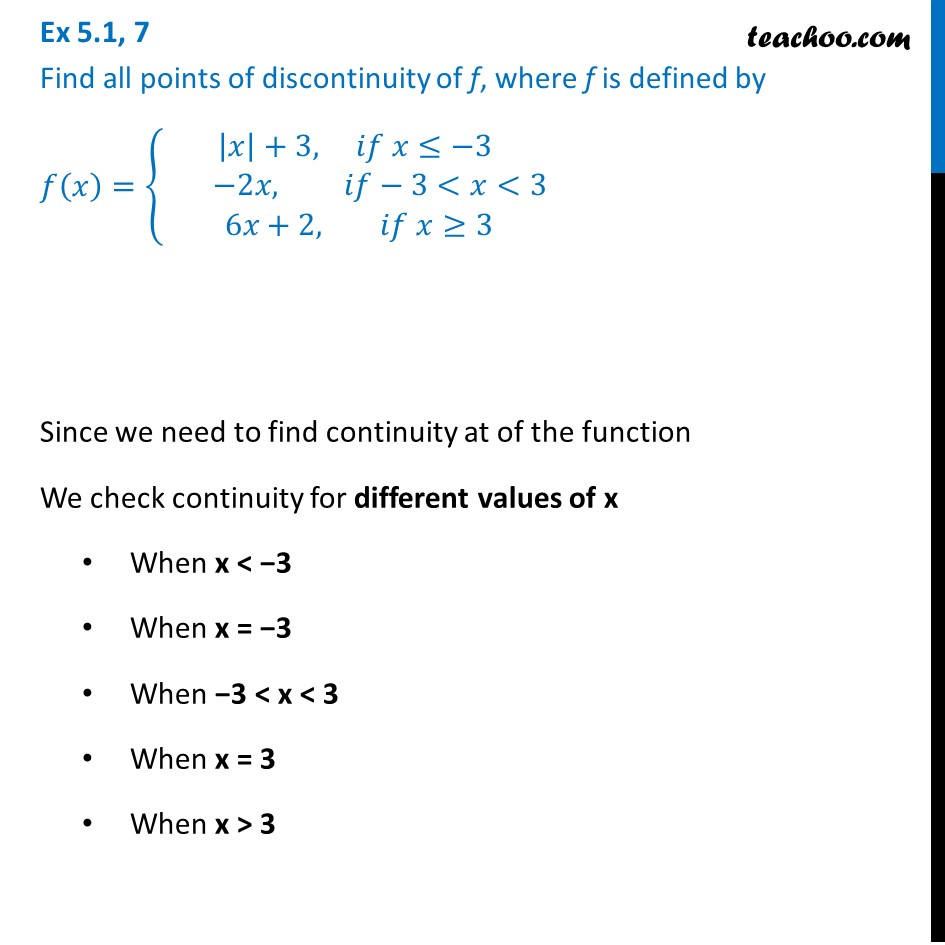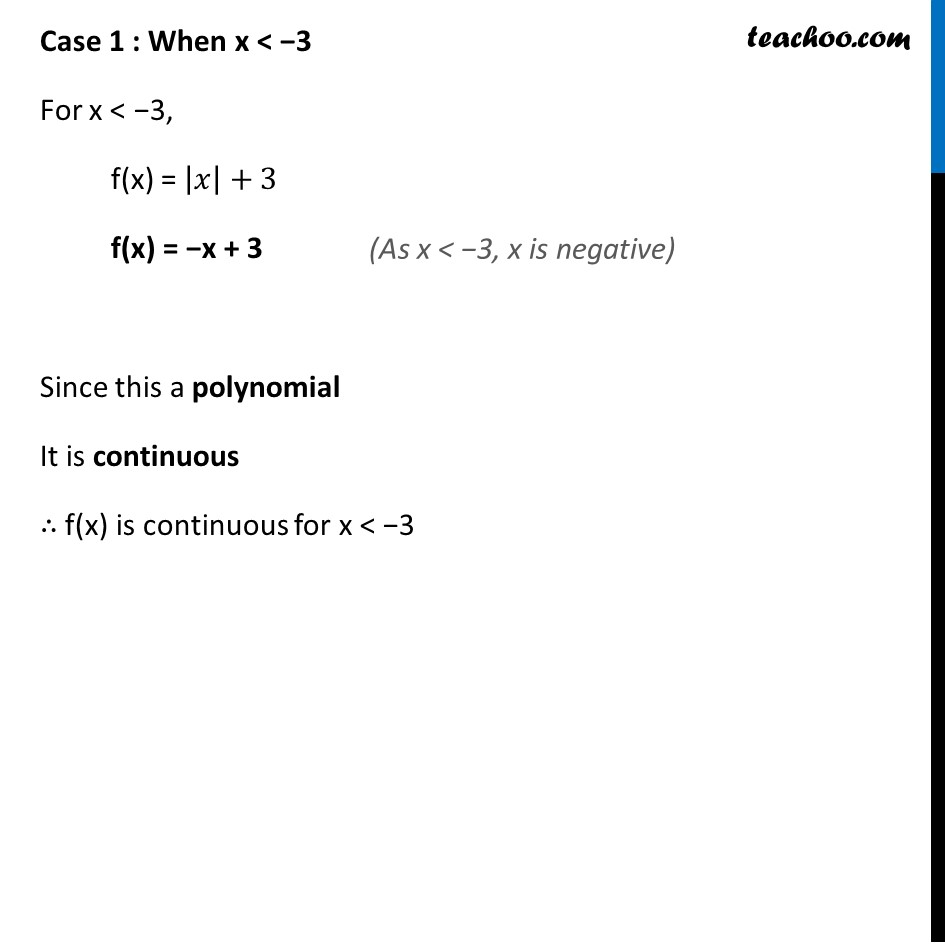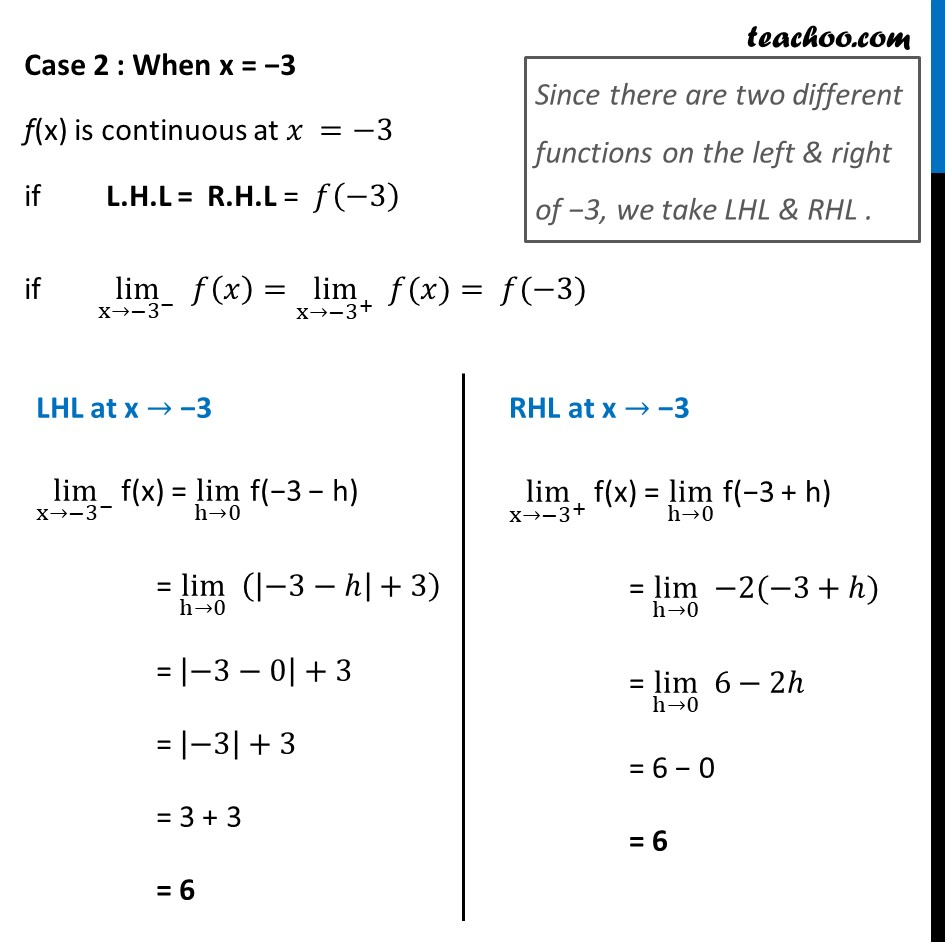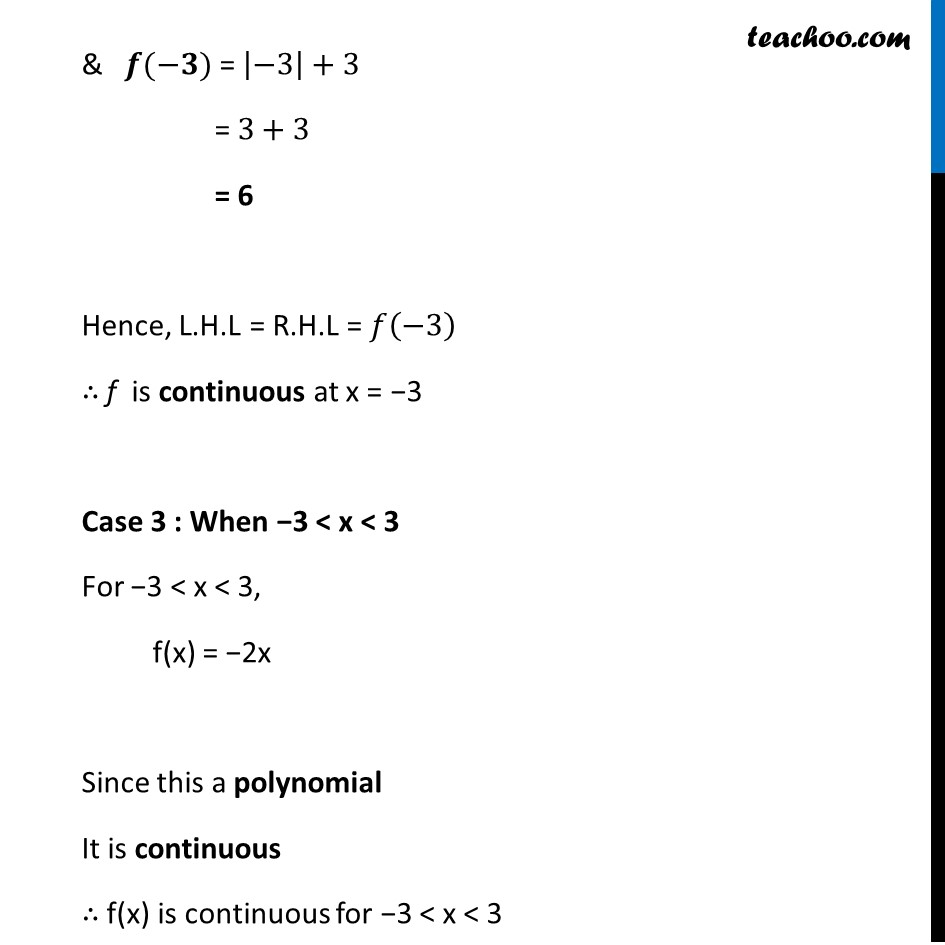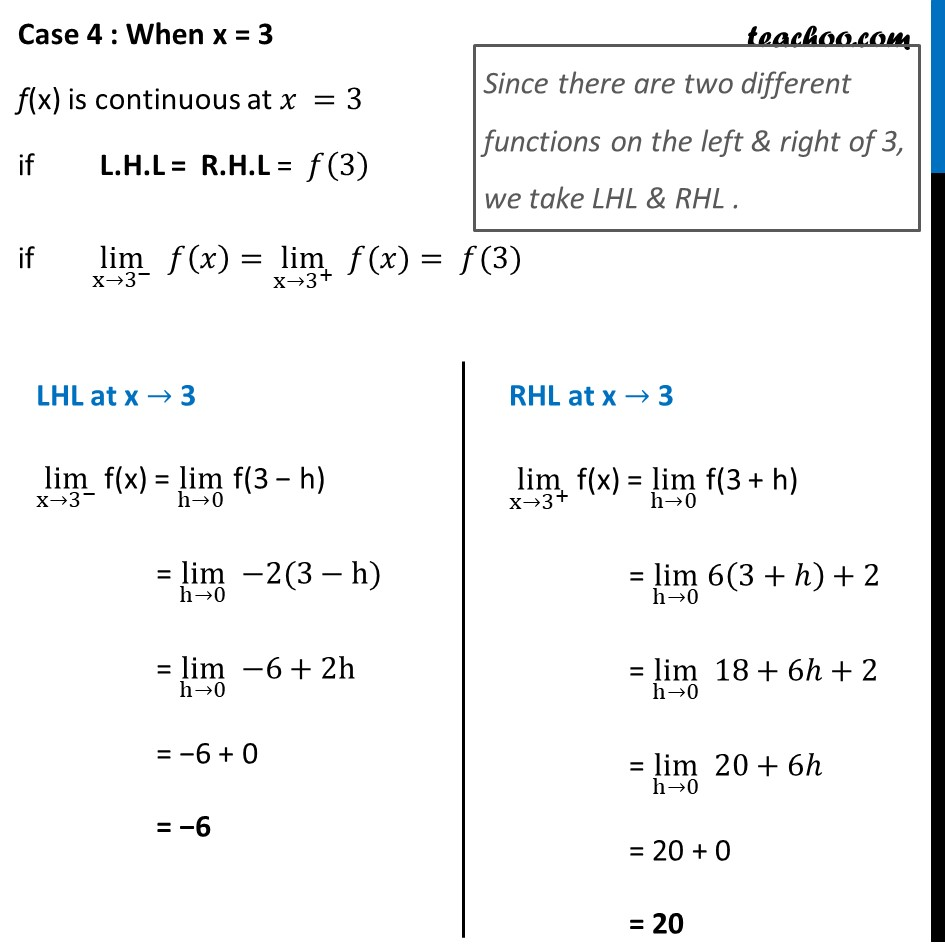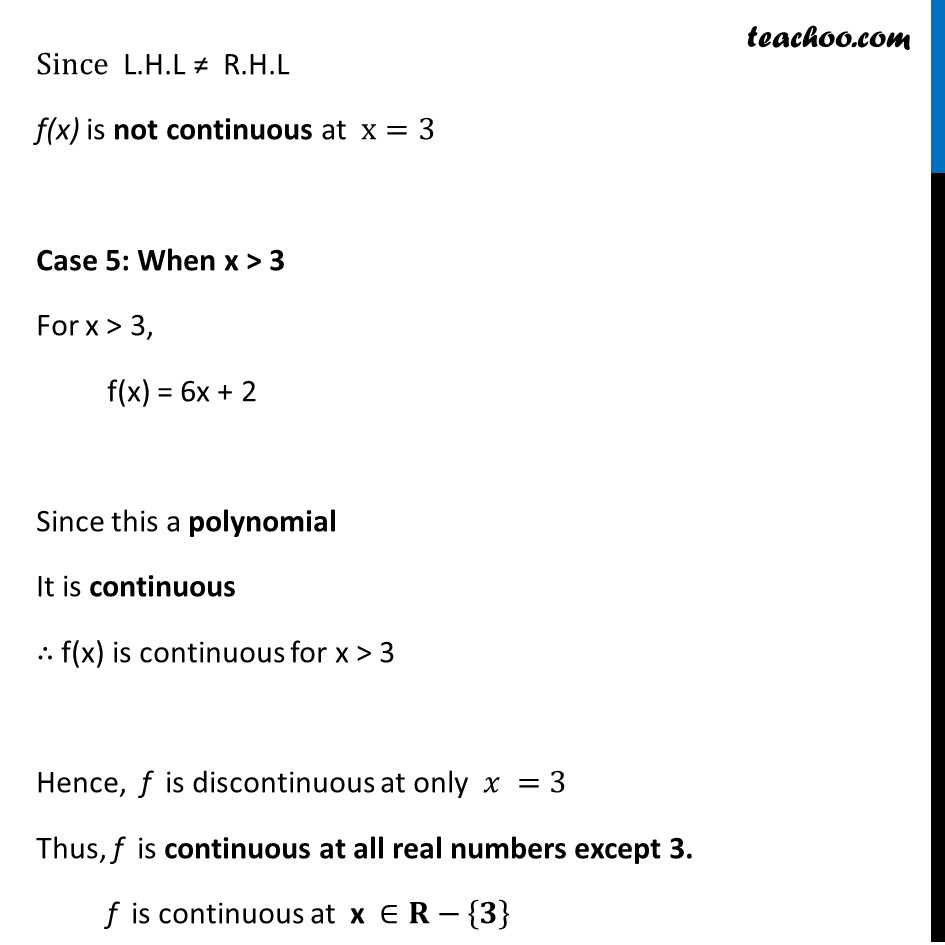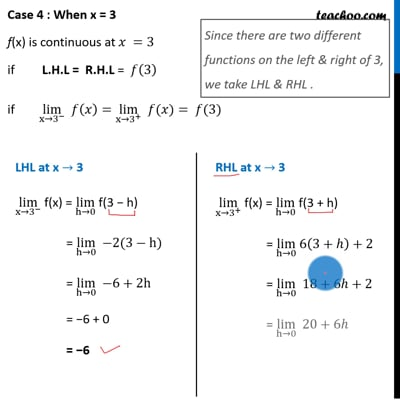This video is only available for Teachoo black users

Solve all your doubts with Teachoo Black (new monthly pack available now!)

### Transcript

Ex 5.1, 7 Find all points of discontinuity of f, where f is defined by 𝑓(𝑥)={█(|𝑥|+3, 𝑖𝑓 𝑥≤−3@ −2𝑥, 𝑖𝑓−3<𝑥<3@ 6𝑥+2, 𝑖𝑓 𝑥≥3)┤ Since we need to find continuity at of the function We check continuity for different values of x When x < −3 When x = −3 When −3 < x < 3 When x = 3 When x > 3 Case 1 : When x < −3 For x < −3, f(x) = |𝑥|+3 f(x) = −x + 3 Since this a polynomial It is continuous ∴ f(x) is continuous for x < −3 (As x < −3, x is negative) Case 2 : When x = −3 f(x) is continuous at 𝑥 =−3 if L.H.L = R.H.L = 𝑓(−3) if lim┬(x→〖−3〗^− ) 𝑓(𝑥)=lim┬(x→〖−3〗^+ ) " " 𝑓(𝑥)= 𝑓(−3) Since there are two different functions on the left & right of −3, we take LHL & RHL . LHL at x → −3 lim┬(x→〖−3〗^− ) f(x) = lim┬(h→0) f(−3 − h) = lim┬(h→0) (|−3−ℎ|+3) = |−3−0|+3 = |−3|+3 = 3 + 3 = 6 RHL at x → −3 lim┬(x→〖−3〗^+ ) f(x) = lim┬(h→0) f(−3 + h) = lim┬(h→0) −2(−3+ℎ) = lim┬(h→0) 6−2ℎ = 6 − 0 = 6 & 𝒇(−𝟑) = |−3|+3 = 3+3 = 6 Hence, L.H.L = R.H.L = 𝑓(−3) ∴ f is continuous at x = −3 Case 3 : When −3 < x < 3 For −3 < x < 3, f(x) = −2x Since this a polynomial It is continuous ∴ f(x) is continuous for −3 < x < 3 Case 4 : When x = 3 f(x) is continuous at 𝑥 =3 if L.H.L = R.H.L = 𝑓(3) if lim┬(x→3^− ) 𝑓(𝑥)=lim┬(x→3^+ ) " " 𝑓(𝑥)= 𝑓(3) Since there are two different functions on the left & right of 3, we take LHL & RHL . LHL at x → 3 lim┬(x→3^− ) f(x) = lim┬(h→0) f(3 − h) = lim┬(h→0) −2(3−h) = lim┬(h→0) −6+2h = −6 + 0 = −6 RHL at x → 3 lim┬(x→3^+ ) f(x) = lim┬(h→0) f(3 + h) = lim┬(h→0) 6(3+ℎ)+2 = lim┬(h→0) 18+6ℎ+2 = lim┬(h→0) 20+6ℎ = 20 + 0 = 20 Since L.H.L ≠ R.H.L f(x) is not continuous at x=3 Case 5: When x > 3 For x > 3, f(x) = 6x + 2 Since this a polynomial It is continuous ∴ f(x) is continuous for x > 3 Hence, f is discontinuous at only 𝑥 =3 Thus, f is continuous at all real numbers except 3. f is continuous at x ∈𝐑−{𝟑}